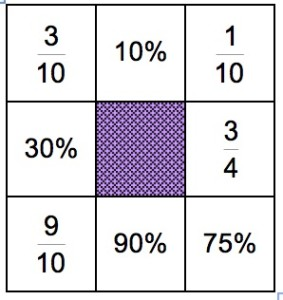The aim is to make square domino loops, like the one in the picture, from 4 dominoes matching the fractions, decimals and percentages. Start with any domino and find 2 other dominoes to match the ends of the first domino.

For example, the [3/10 and 10%] domino matches with 30% at one end and 1/10 at the other end. Then 9/10 matches with 90% and 3/4 with 75% to complete the square. This uses 4 of the dominoes below.

Can you use all 16 dominoes to make 4 domino loops?

Note that in some countries the decimal point is replaced by a decimal comma so that for example $0{\cdot}6$ or $0.6$ is written $0,6$